# Talk:Boltzmann distribution

I think that the current definition of Boltzmann distribution is misleading. The probability of a microsate, say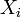$X_i$, is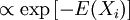$\propto \exp \left[ - E(X_i) \right]$. but a given energy can be degenerate, so I think that it should be written something like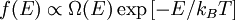$f(E) \propto \Omega(E) \exp \left[ - E/k_B T \right]$,
where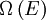$\Omega \left( E \right)$ is the degeneracy of the energy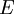$E$; therefore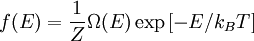$f(E) = \frac{1}{Z} \Omega(E) \exp \left[ -E/k_B T \right]$.